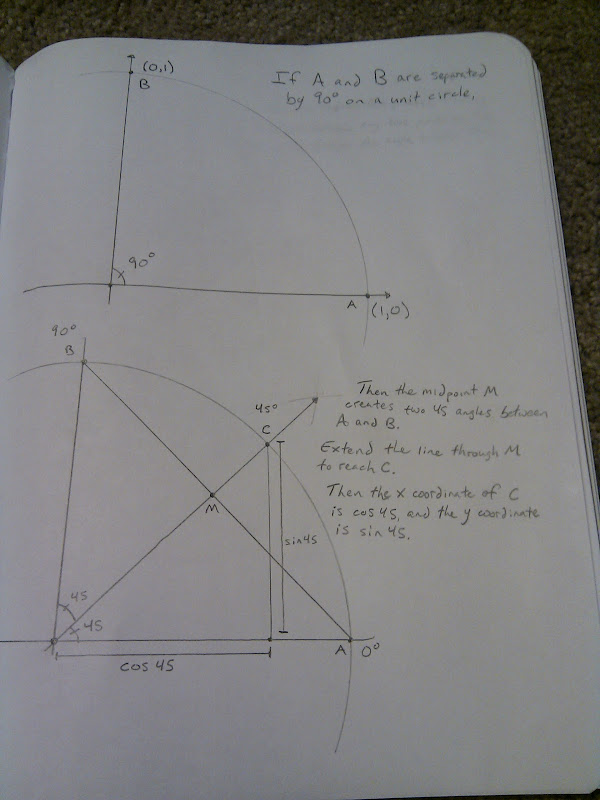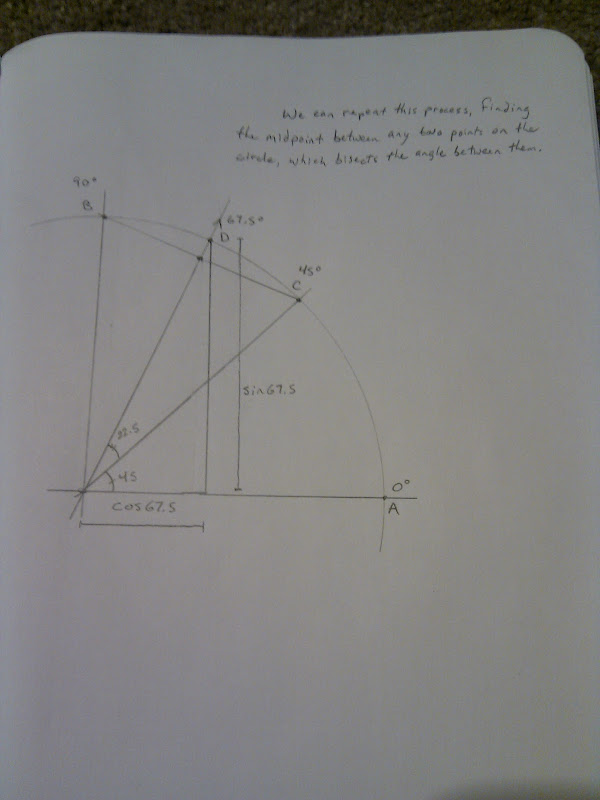## Saturday, June 12, 2010

### Intuitively simple way to calculate sin and cos

A few years ago my brother in law asked how pi is calculated, and we found that it can be done as a reasonably intuitive algorithm, without any advanced math.

Ever since then, I've wondered if sines and cosines could also be calculated simply. Here's what I came up with. Please let me know if you know of a simpler way, where "simpler" means relying on fewer or more elementary mathematical principles.

Sines and cosines let us translate an angle in a right triangle into the length of one of is sides. But what is an angle? Well, on a unit circle, a 45 degree slice cuts off an arc that's 45/360ths the length of the circumference. We can cut that slice in half by finding the midpoint between the two ends of the arc, then extending that line out until it touches the edge of the circle. That slice represents 22.5 degrees. Since we have a point on the unit circle and we know its angle, the point's X and Y coordinates tell us the cosine and sine, respectively, of that angle. (And since the points are on a circle with a radius of 1, we know the hypotenuse of the right triangles is always of length 1).

Here's the same concept in pictures:And here's python code that starts with the two points shown in the pictures, and keeps finding midpoints between them (recursively) until it gets a close enough approximation to any angle you want.

`#!/usr/bin/python"""Released into the public domain, 12 June 2010This program calculates the sine and cosine of an angle in a geometricallyintuitive way.Here's how it works:Start with a unit circle and observe that the points (1,0) and (0,1) on thecircumference of the circle are separated by 90 degrees.The midpoint between those two points, (0.5, 0.5), bisects that 90degree angle, forming two 45 degree angles.  We can extend the line fromthe origin through (0.5, 0.5) until it reaches the circumference of the unitcircle (that is, until its distance from the origin is 1), and we get(0.707, 0.707).  Thus we know that the cosine and sine of 45 degrees are both0.707.Likewise, we can find the cosine and sine of 22.5 degrees by takingthe midpoint between (0.707, 0.707) and (1,0), then scaling the line untilit reaches the circumference.  And we can find the cosine and sine of67.5 degrees by taking the midpoint between (0.707, 0.707) and (0, 1).We can continue to bisect angles until we get arbitrarily close to anyangle we choose.  The allowable angular error is set below as MAX_THETA_ERROR.Note that this method is agnostic to the angular units we use.  If we start offby telling the program that (0,1) and (1,0) are separated by an angle of 90,then the program will return a result measured in degrees.If instead we say that (0,1) and (1,0) are separated by pi/2, we'll get aresult in radians."""import mathMAX_THETA_ERROR=0.01def cos_sin(theta, x1,y1,theta1, x2,y2,theta2):  """cos_sin returns the (cosine,sine) of a value within MAX_THETA_ERROR  units of theta, given two points (x1,y1) and (x2,y2) that live  at angular posisions theta1 and theta2 on the unit circle.  theta,  theta1 and theta2 must all have the same kind of angular unit (degrees,  radians, etc.), but this function doesn't care what that unit is or  how many of them make up a circle.    theta2 must be greater than theta1, and (x1,y1) and (x2,y2) must be  points on the circumference of the unit circle, less than 180 degrees  apart.  for example, cos_sin(30.0, 0.0,1.0,0.0, 1,0,0.0,90.0) returns  (0.866089312575, 0.499889290387), which are the cosine and sine of 30.  """  # Uncomment this to see the successive approximations  #print "target: %.2f.  (%.2f, %.2f)=%.2f (%.2f,%.2f)=%.2f" % \  #  (theta, x1, y1, theta1, x2, y2, theta2)  # if one of the points is close enough to theta, we're done!  if (abs(theta1 - theta) < MAX_THETA_ERROR):    return (x1,y1)  if (abs(theta2 - theta) < MAX_THETA_ERROR):    return (x2,y2)  # the midpoint is just the average of the two points  x_midpoint = (x1 + x2) / 2.0;  y_midpoint = (y1 + y2) / 2.0;  # scale (x_midpoint,y_midpoint) by 1/midpoint_length to get a point  # exactly 1 unit away from the origin  midpoint_length = math.sqrt(x_midpoint*x_midpoint + y_midpoint*y_midpoint)  x_midpoint = x_midpoint / midpoint_length  y_midpoint = y_midpoint / midpoint_length  # the midpoint bisects the angle between the two points  theta_midpoint = (theta1 + theta2) / 2.0;  # figure out which side of the midpoint our target value theta lives on,  # and bisect the extended midpoint with one of the original points to get  # closer to the target  if (theta >= theta_midpoint):    return cos_sin(theta, x_midpoint, y_midpoint, theta_midpoint, x2, y2, theta2)  else:    return cos_sin(theta, x1, y1, theta1, x_midpoint, y_midpoint, theta_midpoint)angle = 30.0(cosine, sine) = cos_sin(angle, 1.0,0.0,0.0,  0.0,1.0,90.0)print "The cosine of %.2f is %.2f" % (angle, cosine)print "The sine of %.2f is %.2f" % (angle, sine)`